# HSPT Math : Concepts

## Example Questions

### Example Question #665 : Hspt Mathematics

Fill in the blank with the proper sign.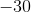__________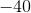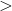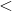Explanation:

In order to solve this problem, we will use a number line. Numbers to the left of zero on the line are less than numbers to the right.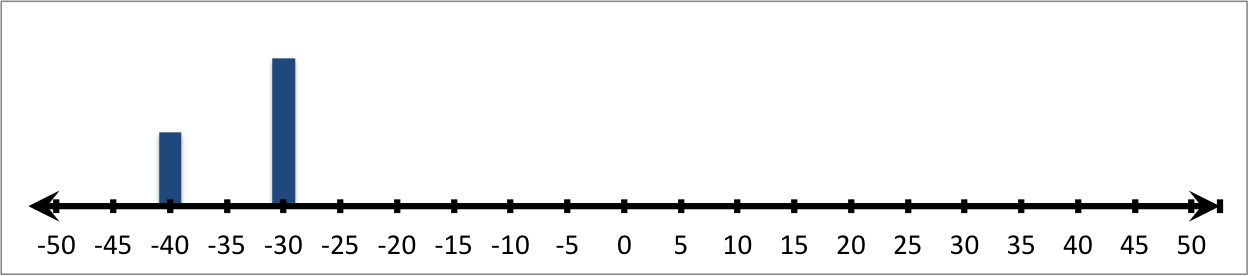Therefore: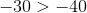### Example Question #31 : Understand An Inequality On A Number Line: Ccss.Math.Content.6.Ns.C.7a

Fill in the blank with the proper sign.__________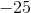Explanation:

In order to solve this problem, we will use a number line. Numbers to the left of zero on the line are less than numbers to the right.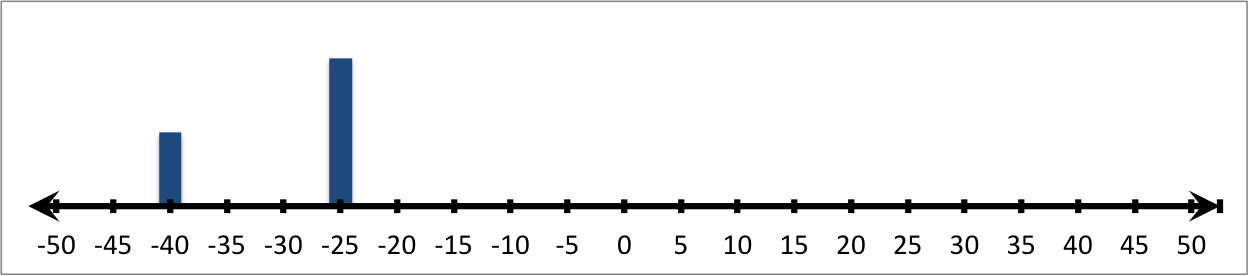Therefore: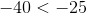### Example Question #32 : Understand An Inequality On A Number Line: Ccss.Math.Content.6.Ns.C.7a

Fill in the blank with the proper sign.__________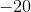Explanation:

In order to solve this problem, we will use a number line. Numbers to the left of zero on the line are less than numbers to the right.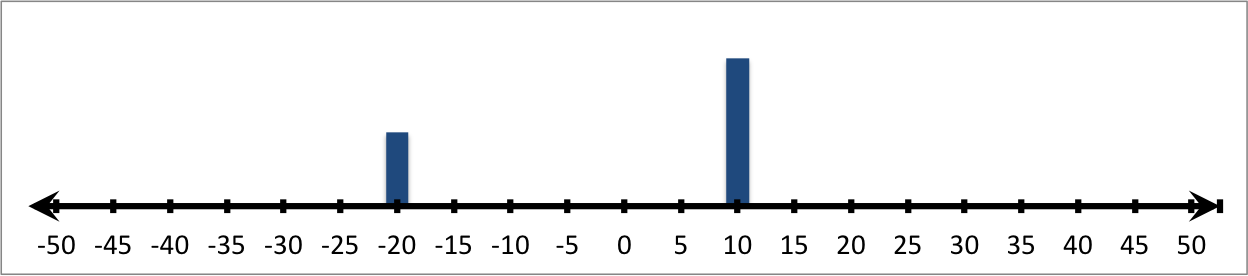Therefore: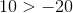### Example Question #33 : Understand An Inequality On A Number Line: Ccss.Math.Content.6.Ns.C.7a

Fill in the blank with the proper sign.__________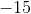Explanation:

In order to solve this problem, we will use a number line. Numbers to the left of zero on the line are less than numbers to the right.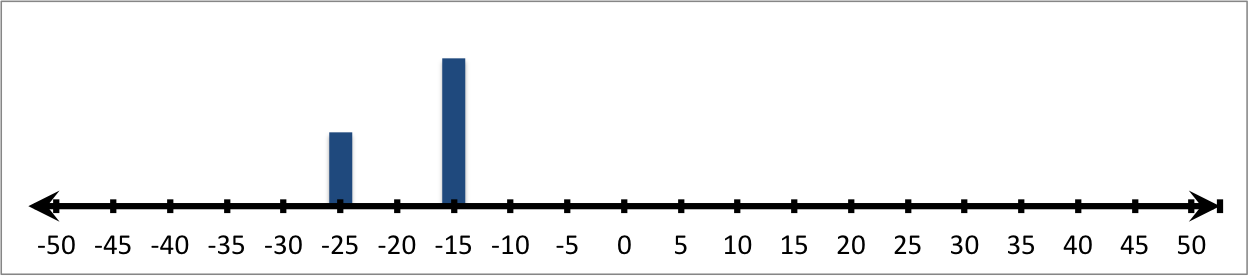Therefore: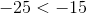### Example Question #34 : Understand An Inequality On A Number Line: Ccss.Math.Content.6.Ns.C.7a

Fill in the blank with the proper sign.__________Explanation:

In order to solve this problem, we will use a number line. Numbers to the left of zero on the line are less than numbers to the right.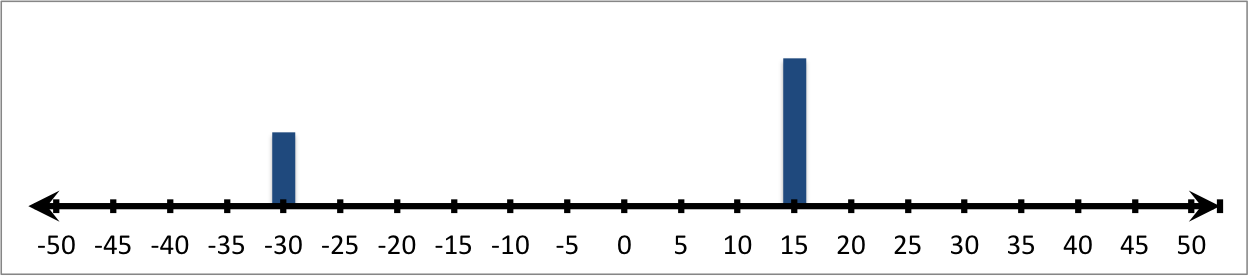Therefore: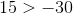### Example Question #661 : Hspt Mathematics

Fill in the blank with the proper sign.__________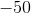Explanation:

In order to solve this problem, we will use a number line. Numbers to the left of zero on the line are less than numbers to the right.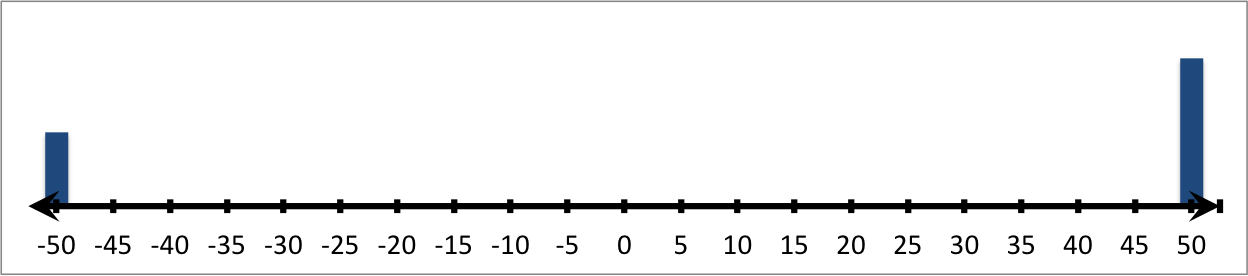Therefore: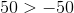### Example Question #36 : Understand An Inequality On A Number Line: Ccss.Math.Content.6.Ns.C.7a

Fill in the blank with the proper sign.__________Explanation:

In order to solve this problem, we will use a number line. Numbers to the left of zero on the line are less than numbers to the right.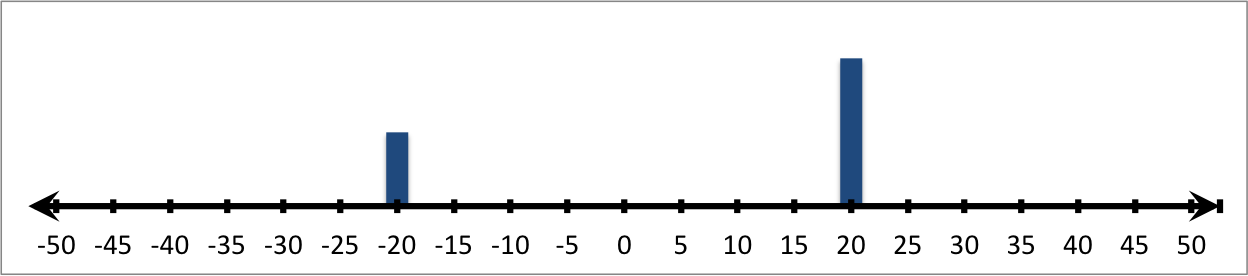Therefore: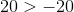### Example Question #37 : Understand An Inequality On A Number Line: Ccss.Math.Content.6.Ns.C.7a

Fill in the blank with the proper sign.__________Explanation:

In order to solve this problem, we will use a number line. Numbers to the left of zero on the line are less than numbers to the right.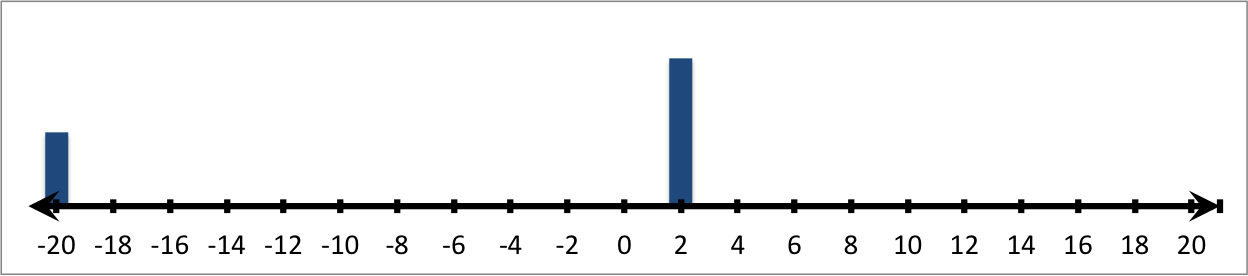Therefore: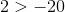### Example Question #38 : Understand An Inequality On A Number Line: Ccss.Math.Content.6.Ns.C.7a

Fill in the blank with the proper sign.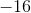__________Explanation:

In order to solve this problem, we will use a number line. Numbers to the left of zero on the line are less than numbers to the right.Therefore: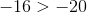### Example Question #39 : Understand An Inequality On A Number Line: Ccss.Math.Content.6.Ns.C.7a

ill in the blank with the proper sign.__________Explanation:

In order to solve this problem, we will use a number line. Numbers to the left of zero on the line are less than numbers to the right.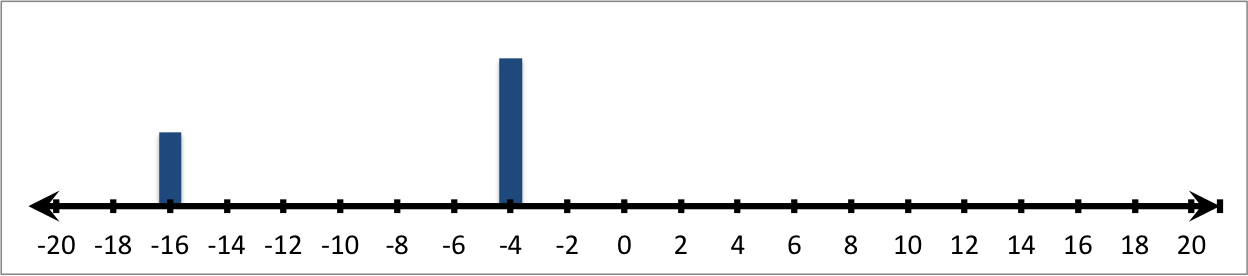Therefore: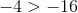### All HSPT Math Resources